# Diode model

The Multisim diode model is based on the original SPICE3 diode model. It contains a number of enhancements, including improved convergence and support for high-injection effects.

See below for details.

Large signal model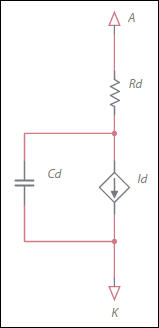In the following equations, Vd is the voltage across source Id. It does not include the drop across Rd.

Static equations

The current through the diode is the sum of the forward current, Ifwd, and reverse current, Irev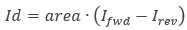Forward current:

The forward current is the sum of the normal and recombination currents: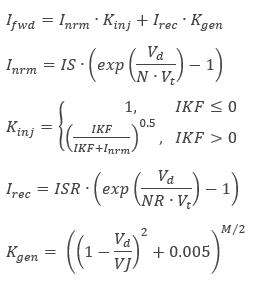With the default parameter values, most of the terms drop out or the factors are set to 1. Therefore the forward current simply degenerates into the normal current, Inrm

Reverse current:

The reverse current is the sum of the high and low reverse currents: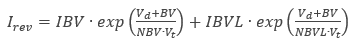Capacitance equations

The diode's non-linear capacitance, Cd, is the sum of the diffusion capacitance: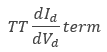and the junction capacitance.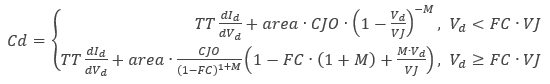Temperature dependent parameters

The following parameters are functions of temperature. T is the operating temperate and TNOM is the nominal (or measured temperature). T and TNOM can be adjusted in a number of ways.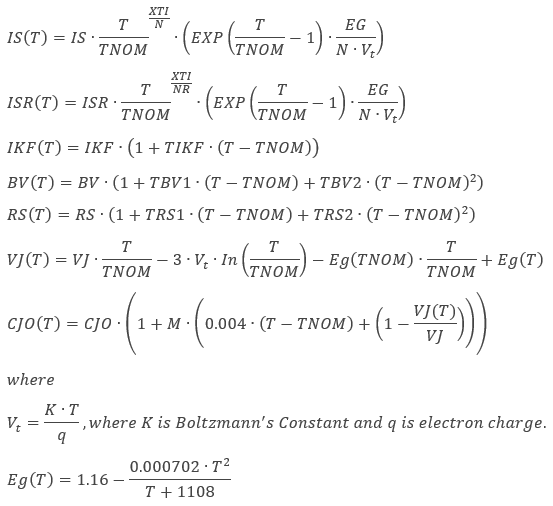Noise model

The diode has a thermal noise generator,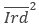, as a result of the series ohmic resistance, and the shot and flicker noise generators, collectively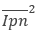, as a result of the PN junction.

Ohmic resistance noise: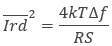Shot and flicker noise: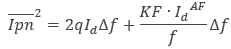References

1. G. Massobrio and P. Antognetti, Semiconductor Device Modeling with SPICE, 2nd edition, McGraw-Hill, 1993.
2. A. Vladimirescu, The SPICE Book, Wiley, 1994.